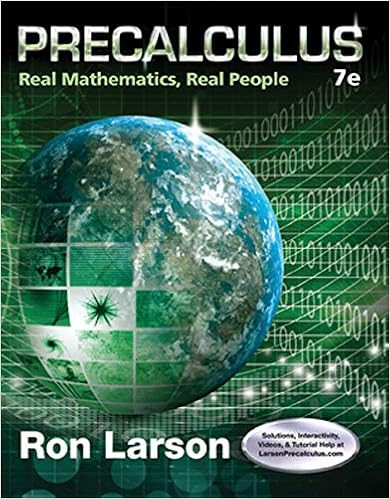# Now do exercises 2334 u 5 v intervals of real numbers

• Notes
• 84
• 100% (3) 3 out of 3 people found this document helpful

This preview shows page 6 - 8 out of 84 pages.

##### We have textbook solutions for you!
The document you are viewing contains questions related to this textbook.The document you are viewing contains questions related to this textbook.
Chapter 2 / Exercise 99
Precalculus: Real Mathematics, Real People
LarsonExpert Verified
Now do Exercises 23–34 U 5 V Intervals of Real Numbers Retailers often have a sale for a certain interval of time. Between 6 A . M . and 8 A . M . you get a 20% discount. A bounded or finite interval of real numbers is the set of real numbers that are between two real numbers, which are called the endpoints of the interval. The endpoints may or may not belong to an interval. Interval notation is used to represent intervals of real numbers. In interval notation, parentheses are used to indicate that the endpoints do not belong to the interval and brackets indicate that the endpoints do belong to the interval. The following box shows the four types of finite intervals for two real numbers a and b , where a is less than b . Finite Intervals Verbal Description Interval Notation Graph The set of real numbers ( a , b ) between a and b The set of real numbers [ a , b ] between a and b inclusive The set of real numbers greater ( a , b ] than a and less than or equal to b The set of real numbers greater [ a , b ) than or equal to a and less than b a b a b a b a b 0 1 2 3 4 5 6 The interval [2, 5) 0 1 2 3 4 5 6 Figure 1.11 Note how the parentheses and brackets are used on the graph and in the interval nota- tion. It is also common to draw the graph of an interval of real numbers using an open cir- cle for an endpoint that does not belong to the interval and a closed circle for an endpoint that belongs to the interval. For example, see the graphs of the interval [2, 5) in Fig. 1.11. In this text, graphs of intervals will be drawn with parentheses and brackets so that they agree with interval notation.
##### We have textbook solutions for you!
The document you are viewing contains questions related to this textbook.The document you are viewing contains questions related to this textbook.
Chapter 2 / Exercise 99
Precalculus: Real Mathematics, Real People
LarsonExpert Verified
1-7 1.1 The Real Numbers 7 E X A M P L E 4 Interval notation for finite intervals Write the interval notation for each interval of real numbers and graph the interval. a) The set of real numbers greater than 3 and less than or equal to 5 b) The set of real numbers between 0 and 4 inclusive c) The set of real numbers greater than or equal to 1 and less than 4 d) The set of real numbers between 2 and 1 Solution a) The set of real numbers greater than 3 and less than or equal to 5 is written in interval notation as (3, 5] and graphed in Fig. 1.12. 1 2 3 4 5 6 The interval (3, 5] Figure 1.12 1 1 0 2 3 4 5 The interval [0, 4] Figure 1.13 Some sales never end. After 8 A . M . all merchandise is 10% off. An unbounded or infinite interval of real numbers is missing at least one endpoint. It may extend infi- nitely far to the right or left on the number line. In this case the infinity symbol is used as an endpoint in the interval notation. Note that parentheses are always used next to or in interval notation, because is not a number. It is just used to indi- cate that there is no end to the interval. The following box shows the five types of infi- nite intervals for a real number a . c) The set of real numbers greater than or equal to 1 and less than 4 is written in interval notation as [ 1, 4) and graphed in Fig. 1.14.
•••